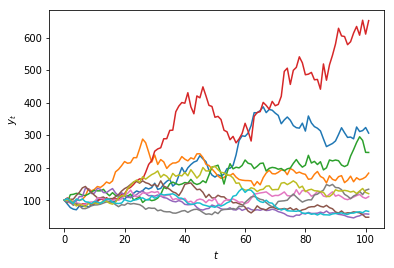Steve Phelps

# A Simple Random Walk¶

• Imagine a board-game in which we move a counter either up or down on an infinite grid based on the flip of a coin.

• We start in the center of the grid at position $y_1 = 0$.

• Each turn we flip a coin. If it is heads we move up one square, otherwise we move down.

• How will the counter behave over time? Let's simulate this in Python.

• First we create a variable $y$ to hold the current position

In :
y = 0


# Movements as Bernoulli trials¶

• Now we will generate a Bernoulli sequence representing the moves
• Each movement is an i.i.d. discrete random variable $\epsilon_t$ distributed with $p(\epsilon_t = 0) = \frac{1}{2}$ and $p(\epsilon_t = 1) = \frac{1}{2}$.
• We will generate a sequence $( \epsilon_1, \epsilon_2, \ldots, \epsilon_{t_{max}} )$ such movements, with $t_{max} = 100$.
• The time variable is also discrete, hence this is a discrete-time model.
• This means that time values can be represented as integers.
In :
import numpy as np
from numpy.random import randint

max_t = 100
movements = randint(0, 2, size=max_t)
print(movements)

[0 0 1 0 1 0 0 1 1 0 0 0 1 0 0 1 0 1 1 0 1 1 0 1 1 0 0 1 0 1 0 1 0 1 1 0 1
1 0 0 0 0 1 0 0 1 1 1 1 0 0 1 1 1 0 0 0 1 0 1 0 0 0 1 0 0 0 1 0 0 0 0 0 1
1 0 0 0 1 1 0 0 1 1 0 1 1 1 0 0 0 0 1 0 0 1 0 0 1 0]


# An integer random-walk in Python¶

• Each time we move the counter, we move it in the upwards direction if we flip a 1, and downwards for a 0.
• So we add 1 to $y_t$ for a 1, and subtract 1 for a $0$.
In :
import numpy as np
import matplotlib.pyplot as plt
from numpy.random import randint, normal, uniform
%matplotlib inline
max_t = 100
movements = randint(0, 2, size=max_t)
y = 0
values = [y]
for movement in movements:
if movement == 1:
y = y + 1
else:
y = y - 1
values.append(y)
plt.xlabel('t')
plt.ylabel('y')
ax = plt.plot(values)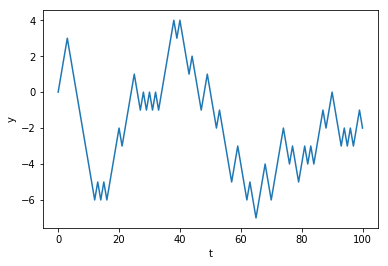# A random-walk as a cumulative sum¶

• Notice that the value of $y_t$ is simply the cumulative sum of movements randomly chosen from $-1$ or $+1$.
• So if $p(\epsilon = -1) = \frac{1}{2}$ and $p(\epsilon = +1) = \frac{1}{2}$ then
• We can define our game as a simple stochastic process : $y_t = \sum_{t=1}^{t_{max}} \epsilon_t$
• We can use numpy's where() function to replace all zeros with $-1$.
In :
t_max = 100
random_numbers = randint(0, 2, size=t_max)
steps = np.where(random_numbers == 0, -1, +1)
y = 0
values = 
for step in steps:
y = y + step
values.append(y)
plt.xlabel('t')
plt.ylabel('y')
ax = plt.plot(values)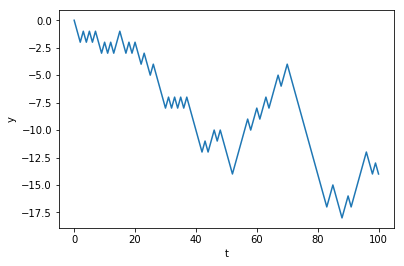# A random-walk using arrays¶

• We can make our code more efficient by using the cumsum() function instead of a loop.
• This way we can work entirely with arrays.
• Remember that vectorized code can be much faster than iterative code.
In :
# Vectorized random-walk with arrays to improve efficiency
t_max = 100
random_numbers = randint(0, 2, size=t_max)
steps = np.where(random_numbers == 0, -1, +1)
values = np.cumsum(steps)
plt.xlabel('t')
plt.ylabel('y')
ax = plt.plot(values)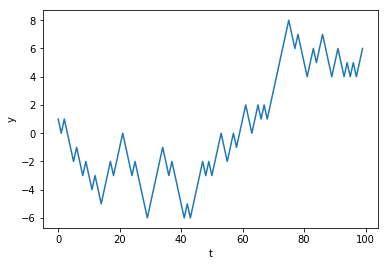## Using concatenate to prepend the initial value¶

• If we want to include the initial position $y_0 = 0$, we can concatenate this value to the computed values from the previous slide.

• The numpy.concatenate() function takes a single argument containing a sequence of arrays, and returns a new array which contains all values in a single array.

In :
plt.plot(np.concatenate((, values)))
plt.xlabel('$t$')
plt.ylabel('$y_t$')
plt.show()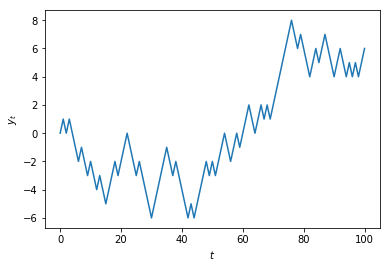# Multiple realisations of a stochastic process¶

• Because we are making use of random numbers, each time we execute this code we will obtain a different result.

• In the case of a random-walk, the result of the simulation is called a path.

• Each path is called a realisation of the model.

• We can generate multiple paths by using a 2-dimensional array (a matrix).

• Suppose we want $n= 10$ paths.

• In Python we can pass two values for the size argument in the randint() function to specify the dimensions (rows and columns):

In :
t_max = 100
n = 10
random_numbers = randint(0, 2, size=(t_max, n))
steps = np.where(random_numbers == 0, -1, +1)


# Using cumsum()¶

We can then tell cumsum() to sum the rows using the axis argument:

In :
values = np.cumsum(steps, axis=0)
values = np.concatenate((np.matrix(np.zeros(n)), values), axis=0)
plt.xlabel('$t$')
plt.ylabel('$y_t$')
ax = plt.plot(values)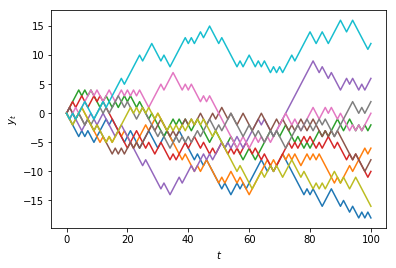# Multiplicative Random Walks¶

• The series of values we have looked at do not closely resememble how security prices change over time.

• In order to obtain a more realistic model of how prices change over time, we need to multiply instead of add.

• Let $r_t$ denote an i.i.d. random variable distributed $r_t \sim N(0, \sigma^2)$

• Define a strictly positive intitial value $y_0 \in \mathbf{R}$; e.g. $y_0 = 10$.

• Subsequent values are given by $y_t = y_{t-1} \times (1 + r_t)$

• We can write this as a cumulative product:

$y_t = y_0 \times \Pi_{t=1}^{t_{max}} \epsilon_t$

# Using cumprod()¶

In :
initial_value = 100.0
random_numbers = normal(size=t_max) * 0.005
multipliers = 1 + random_numbers
values = initial_value * np.cumprod(multipliers)
plt.xlabel('$t$')
plt.ylabel('$y_t$')
ax = plt.plot(np.concatenate(([initial_value], values)))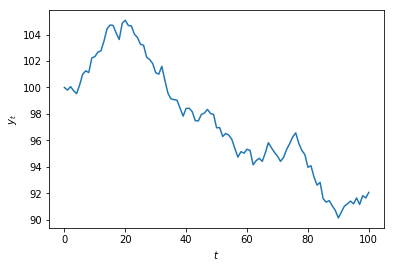# Random walk variates as a time-series¶

• Now let's plot the random perturbations over time
In :
plt.xlabel('$t$')
plt.ylabel('$r_t$')
ax = plt.plot(random_numbers)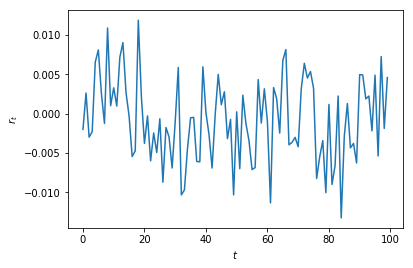# Gross returns¶

• If we take $100 \times \epsilon_t$, then these represent the percentage changes in the value at discrete time intervals.

• If the values represent prices that have been adjusted to incorporate dividends, then the multipliers are called simple returns.

• The gross return is obtained by adding 1.

In :
plt.xlabel('$t$')
plt.ylabel('$r_t$')
ax = plt.plot(random_numbers + 1)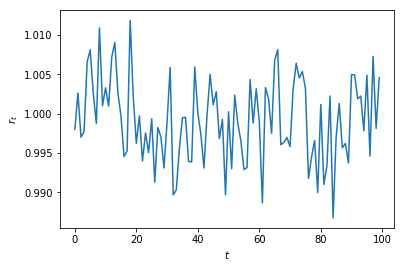# Continuously compounded, or log returns¶

• A simple return $R_t$ is defined as

$R_t = (y_t - y_{t-1}) / y_{t-1} = y_{t} / y_{t-1} - 1$

where $y_t$ is the adjusted price at time $t$.

• The gross return is $R_t + 1$

• A continuously compounded return $r_t$, or log-return, is defined as:

$r_t = \log(y_t / y_{t-1}) = \log(y_t) - \log(y_{t-1})$

• In Python:
In :
from numpy import diff, log

prices = values
log_returns = diff(log(prices))


# Aggregating returns¶

• Simple returns aggregate across assets- the return on a portfolio of assets is the weighted average of the simple returns of its individual securities.

• Log returns arregate across time.

• If return in year one is $r_1 = \log(p_1 / p_0) = \log(p_1) - \log(p_0)$

• and return in year two is $r_2 = \log(p_2 / p_1) = \log(p_2) - \log(p_1)$,
• then return over two years is $r_1 + r_2 = \log(p_2) - \log(p_0)$

# Converting between simple and log returns¶

• A simple return $R_t$ can be converted into a log-return $r_t$:

$r_t = \log(R_t + 1)$

# Comparing simple and log returns¶

• For small values of $r_t$ then $R_t \approx r_t$.

• We can examine the error for larger values:

In :
simple_returns = np.arange(-0.75, +0.75, 0.01)
log_returns = np.log(simple_returns + 1)
plt.xlim([-1.5, +1.5])
plt.ylim([-1.5, +1.5])
plt.plot(simple_returns, log_returns)
x = np.arange(-1.5, 1.6, 0.1)
plt.xlabel('R')
plt.ylabel('r')
ax = plt.plot(x, x)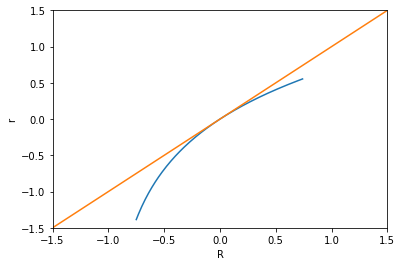# A discrete multiplicative random walk with log returns¶

• Let $r_t$ denote a random i.i.d. variable distributed $r_t \sim N(0, \sigma^2)$

• Then $y_t = y_0 \times \operatorname{exp}(\sum_{t=1}^{t_{max}} r_t)$

In :
from numpy import log, exp, cumsum

t_max = 100
volatility = 1e-2
initial_value = 100.
r = normal(size=t_max) * np.sqrt(volatility)
y = initial_value * exp(cumsum(r))
plt.xlabel('$t$')
plt.ylabel('$y_t$')
ax = plt.plot(np.concatenate(([initial_value], y)))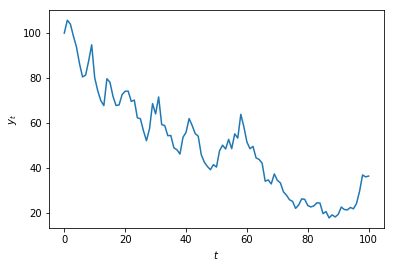# Multiple realisations of a multiplicative random-walk¶

• Let's generate $n=10$ realisations of this process:
In :
def random_walk(initial_value = 100, n = 10,
t_max = 100, volatility = 0.005):
r = normal(size=(t_max+1, n)) * np.sqrt(volatility)
return np.concatenate((np.matrix([initial_value] * n),
initial_value * exp(np.cumsum(r, axis=0))))

plt.xlabel('$t$')
plt.ylabel('$y_t$')
ax = plt.plot(random_walk(n=10))